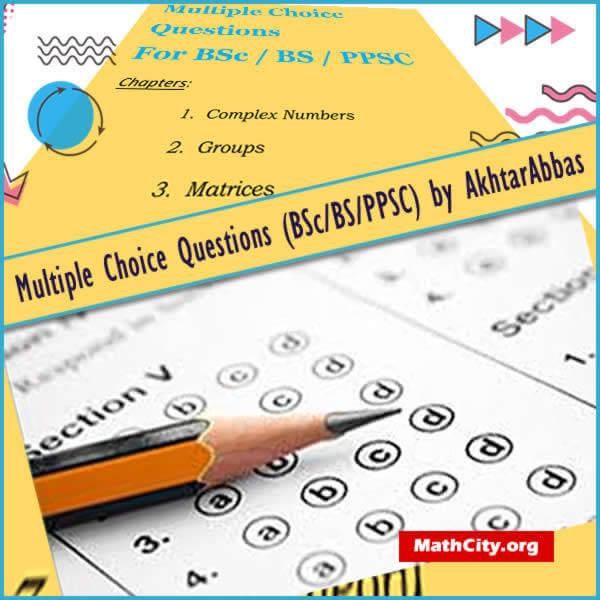# Multiple Choice Questions (BSc/BS/PPSC) by Akhtar AbbasThese notes are made and shared by Mr. Akhtar Abbas. We are really very thankful to him for providing these notes and appreciates his efforts to publish these notes on MathCity.org. Multiple Choice Questions (MCQs) are given in these notes, which might be helpful in BSc, BS or Punjab Public Service Commission (PPSC) exams.

 Name Multiple Choice Questions (BSc/BS/PPSC) Mr. Akhtar Abbas 99 pages PDF (see Software section for PDF Reader) 706 kB

Explicitly MCQs for the following subjects are given.

1. Complex Numbers
2. Groups
3. Matrices
4. System of Linear Equations
5. Determinants
6. Metric Spaces
7. Number Theory
8. Ordinary Differential Equations
9. Infinite Series

Here are few samples:

1. Complex numbers with 0 as real part are called:
1. imaginary numbers
2. pure non real numbers
3. pure imaginary numbers
4. pure complex numbers
2. For any positive integers $a$ and $b$, there exists a positive integer $n$ such that $na > b$ is

called:

1. Archimedean Property
2. Division Algorithm
3. Density Theorem
4. Fundamental Theorem of Arithmetic
5. $(p − 1)! \equiv −1(mod p)$ if and only if
6. $p$ is a prime
7. $p$ is an odd prime
8. $p$ is an odd integer
9. None of these

• notes/multiple-choice-questions-_bsc-bs-ppsc_-akhtar-abbas